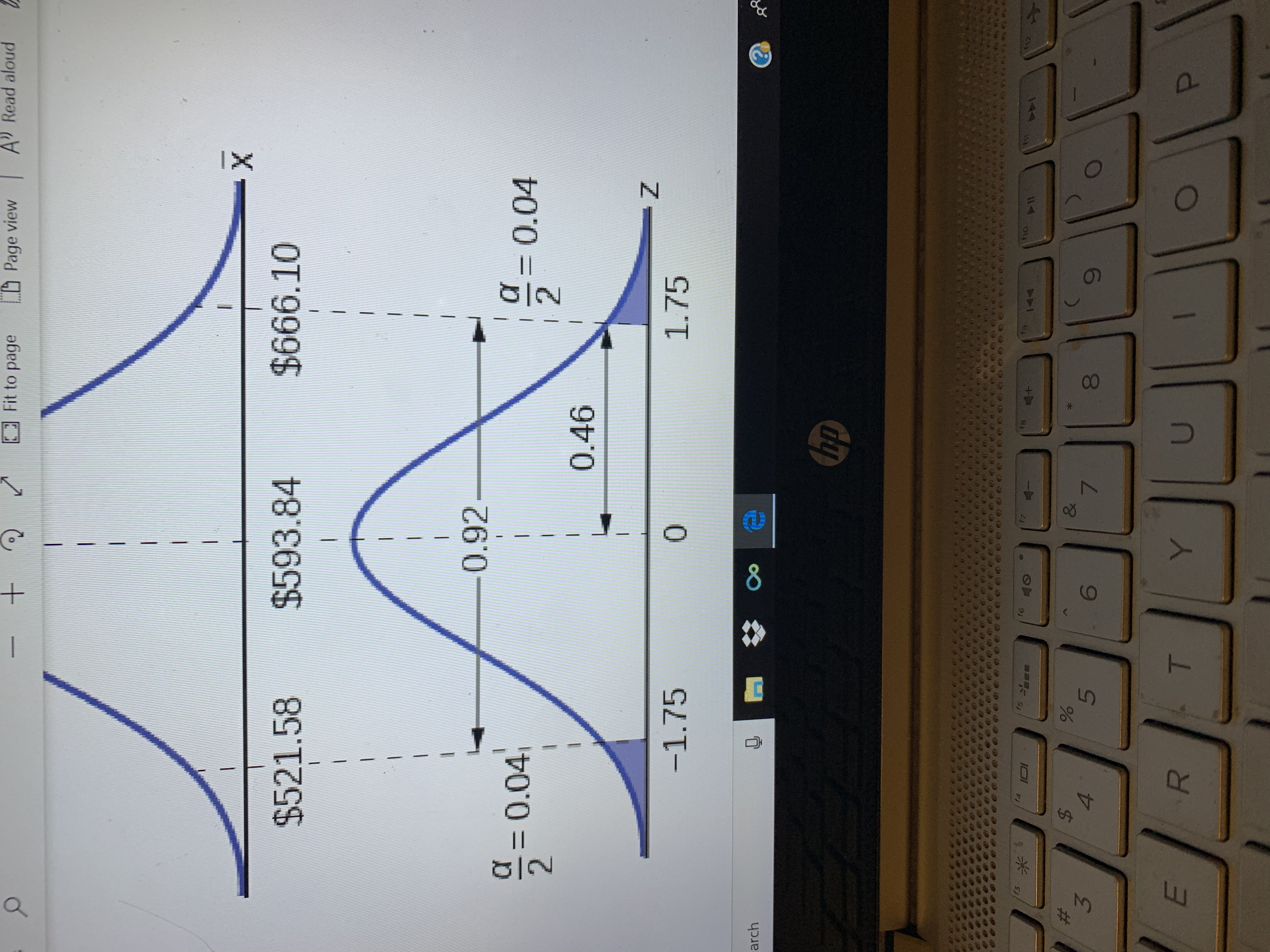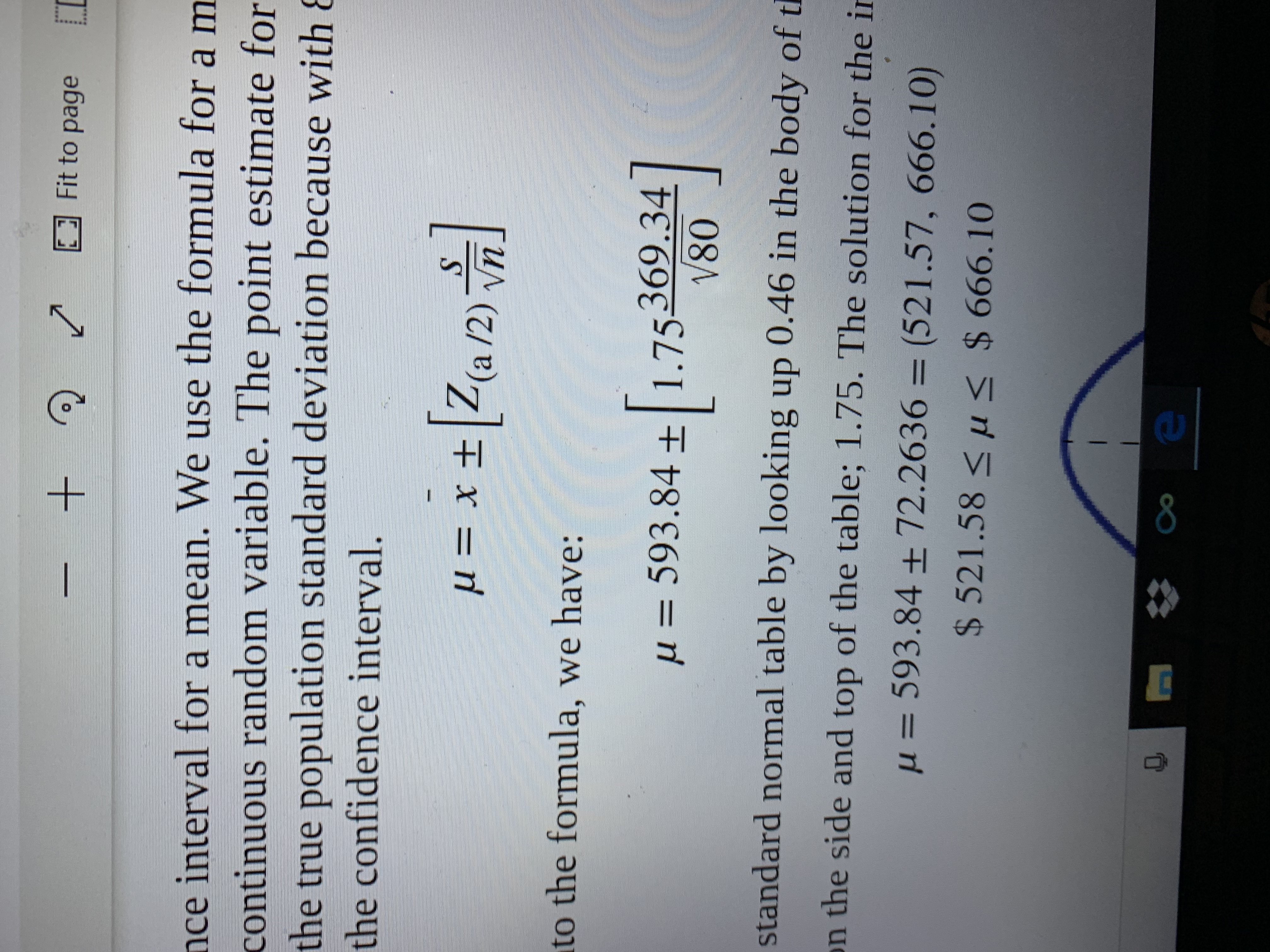# +A Read aloudFit to pagePage view|IX$666.10$593.84$521.580.920.0420.0420.46- Z-1.751.75archhpfafsf6T8hofoASt44$4&357YP008LE +Fit to pagence interval for a mean. We use the formula for a mcontinuous random variable. The point estimate forthe true population standard deviation because with 8the confidence interval.xt(a/2)Vnto the formula, we have:593.84 +1.75369.34V80иstandard normal table by looking up 0.46 in the body of tn the side and top of the table; 1.75. The solution for the in= 593.84 + 72.2636 = (521 .57, 666.10)u$521.58< u$ 666.108

Question
36 views

How did they get the 1.75? Using Confidence intervals. Please explain don’t understand how they used the z table they said they used .46help_outlineImage Transcriptionclose+ A Read aloud Fit to page Page view | I X $666.10$593.84 $521.58 0.92 0.04 2 0.04 2 0.46 - Z -1.75 1.75 arch hp fa fs f6 T8 ho fo ASt 44$ 4 & 3 5 7 Y P 00 8 LE fullscreenhelp_outlineImage Transcriptionclose+ Fit to page nce interval for a mean. We use the formula for a m continuous random variable. The point estimate for the true population standard deviation because with 8 the confidence interval. xt(a/2) Vn to the formula, we have: 593.84 +1.75369.34 V80 и standard normal table by looking up 0.46 in the body of t n the side and top of the table; 1.75. The solution for the in = 593.84 + 72.2636 = (521 .57, 666.10) u $521.58< u$ 666.10 8 fullscreen
check_circle

Step 1

For 92% confiden...

### Want to see the full answer?

See Solution

#### Want to see this answer and more?

Solutions are written by subject experts who are available 24/7. Questions are typically answered within 1 hour.*

See Solution
*Response times may vary by subject and question.
Tagged in

### Hypothesis Testing